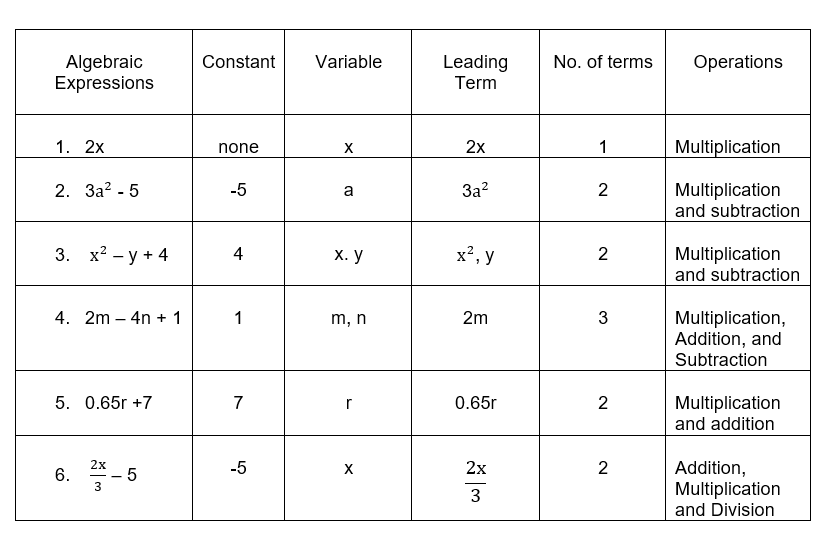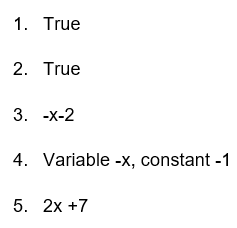# Algebraic Expressions for Grade 7 Worksheet (with answer key + PDF)

An expression in algebraic mathematics is a mathematical statement that consists of regular integers, variables such as x and y, and mathematical operators such as add, subtract, multiply, or divide.

## What is the “Algebraic Expressions Grade 7 Worksheet with answer (PDF)”?

This worksheet will explore some of the methods for solving and understanding the components of an expression that are known as terms those that are created independently and then combined using various operators, such as addition or subtraction.

This worksheet on algebraic expressions helps students with mathematical comprehension. This topic is the key to addressing numerous expansive difficulties. Numerous issues in number theory, geometry, and other fields of analysis can be solved by the use of algebraic expressions. Most mathematical equations are solved using algebra.

## Instructions on how to use the “Algebraic Expressions Grade 7 with answer (PDF).”

Study the concept and examples given and try to solve the given exercises below.

## Conclusion

The content can be mastered by a student if they practice answering a variety of questions that are related to the topic. A worksheet on algebraic expressions is comprised of the application of a variety of mathematical processes, word problems, and geometric interpretations.

If you have any inquiries or feedback, please let us know.

## Algebraic Expressions for Grade 7 Worksheet (with answer key + PDF)

Variables and Constants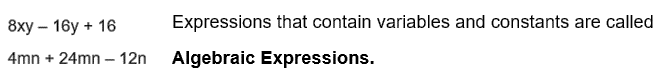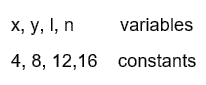When terms have the same algebraic expressions, they are called like terms, and when terms have different algebraic expressions, they are called, unlike terms.

Formation of Expressions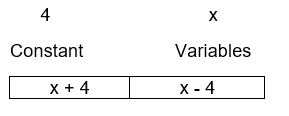Terms of an Expression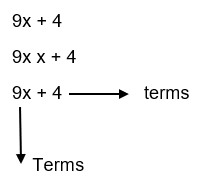Polynomials

Algebraic expressions that contain only one term are called monomials.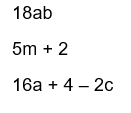Algebraic expressions that contain two terms are called binomials.

Algebraic expressions that contain three terms are called trinomials

1. Complete the table.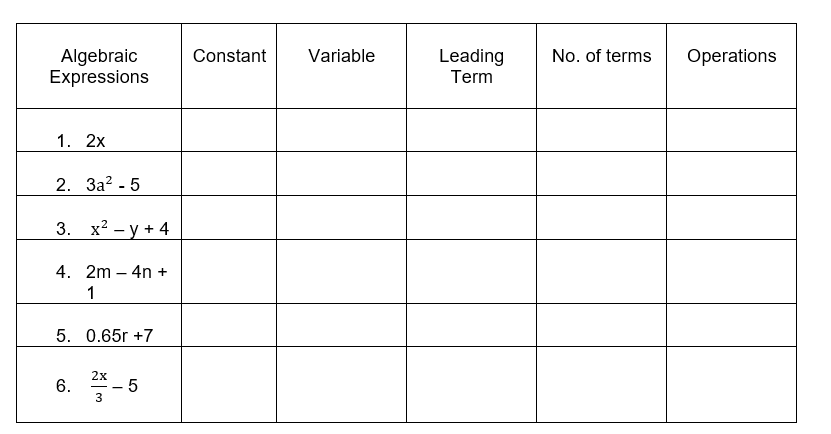## II.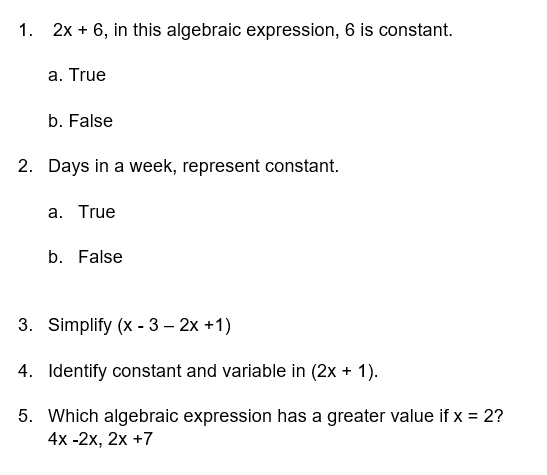1.